Next: Bayes Theorem Up: Statistical Background Previous: Introduction

## Basic Definitions of Notation

In this tutorial we will follow the following definitions of probability:

•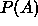probability of event A.
•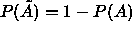probability of non-event A.
•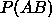probability of simultaneous events A and B.
•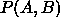joint probability of events A or B.
•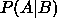probability of event A given event B.
•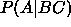probability of event A given events B and C.
•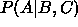probability of event A given events B or C.
•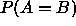probability of equivalence of events A and B.

An event may be defined in any way we wish. An event is always a binary measure but continuous distributions, such as a probability density distribution, can be modelled by defining measures within discrete ranges which may then become infinitessimal. Care should be taken when attempting to interpret probability equations as the notation is only a shorthand for the underlying model and does not contain all the information necessary to understand the range of allowable manipulations for a particular set of data.

Bob Fisher
Fri Mar 28 14:12:50 GMT 1997